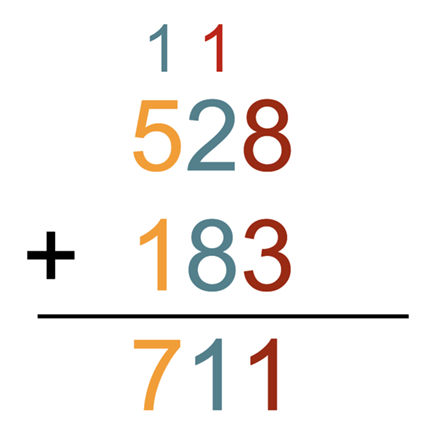# Set 4 Addition, Subtraction & MeasurementYou have lots of strategies for solving addition and subtraction problems.

Have you ever seen someone solve a problem like this?This is called an algorithm. An algorithm is a step-by-step process.

Let’s try an algorithm to solve some problems!